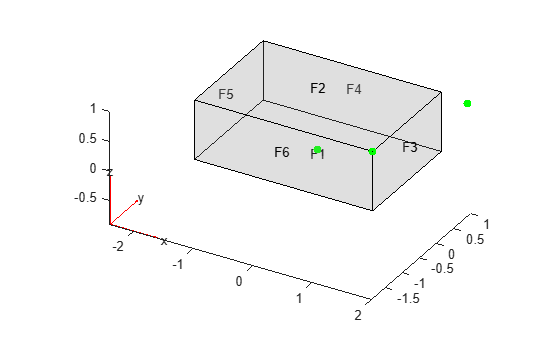# nearestFace

Find faces nearest to specified point

## Syntax

``FaceID = nearestFace(g,Coords)``

## Description

example

````FaceID = nearestFace(g,Coords)` finds faces nearest to the point with the coordinates `Coords`.```

## Examples

collapse all

Find faces of a block nearest to the specified points.

Create a block geometry.

`gm = multicuboid(3,2,1)`
```gm = DiscreteGeometry with properties: NumCells: 1 NumFaces: 6 NumEdges: 12 NumVertices: 8 Vertices: [8x3 double] ```

Plot the geometry with the face labels. Add the points with the coordinates (0 0 0.1), (2 0.9 1), and (1.5 -1 1) to the plot.

```pdegplot(gm,'FaceLabels','on','FaceAlpha',0.2) hold on scatter3([0 2 1.5],[0 0.9 -1],[0.1 1 1],'filled','MarkerFaceColor','g')```Find faces closest to the points with the coordinates (0 0 0.1), (2 0.9 1), and (1.5 -1 1). If several faces are equally close (within the tolerance) to the point, `nearestFace` returns the ID of one of the faces.

`faceIDs = nearestFace(gm,[0 0 0; 2 0.9 1; 1.5 -1 1])`
```faceIDs = 1×3 1 3 6 ```

Find faces of the L-shaped membrane nearest to the specified points.

Create a model and include this geometry. The geometry of the L-shaped membrane is described in the file `lshapeg`.

```model = createpde(); gm = geometryFromEdges(model,@lshapeg)```
```gm = AnalyticGeometry with properties: NumCells: 0 NumFaces: 3 NumEdges: 10 NumVertices: 8 Vertices: [8x2 double] ```

Plot the geometry with the face labels. Add the points with the coordinates (0 0), (1.1 -0.2), and (-0.5 0.5) to the plot.

```pdegplot(gm,'FaceLabels','on') hold on scatter([0 1.1 -0.5],[0 -0.2 0.5],'filled')```Find faces closest to the points with the coordinates (0 0), (1.1 -0.2), and (-0.5 0.5). If several faces are equally close (within the tolerance) to the point, `nearestFace` returns the ID of one of the faces.

`faceIDs = nearestFace(gm,[0 0; 1.1 -0.2; -0.5 0.5])`
```faceIDs = 1×3 2 3 1 ```

## Input Arguments

collapse all

Geometry, specified as a `DiscreteGeometry` or `AnalyticGeometry` object.

Coordinates of the point, specified as a vector of 2 or 3 elements for a 2-D or 3-D geometry, respectively.

Data Types: `double`

## Output Arguments

collapse all

IDs of faces nearest to the specified point, returned as a positive number or a vector of positive numbers.